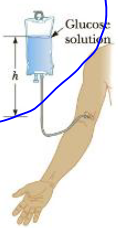Chapter 9, Problem 11P

Chapter
Section
Textbook Problem

A collapsible plastic bag (Fig. F9.11) contains a glucose solution. If the average gauge pressure in the vein is 1.33 × 103 Pa, what must be the minimum height h of the bag to infuse glucose into the vein? Assume the specific gravity of the solution is 1.02.12. A hydraulic jack, has an input piston of area 0.050 m2 and an output piston of area 0.70 m2. How much force on the input piston is required to lift a car weighing 1.2 × 104 N?To determine

The minimum height of the bag to infuse glucose into vein.

Explanation

For the flow of glucose Answer is to flow into the vein, the minimum required gauge pressure of the fluid at the level of needle is equal to the gauge pressure in the vein that is the gauge pressure is Pgauge=PP0=ρghmin and which is rearranged for hmin.

Given info: The gauge pressure in the vein is 1.33×103Pa, specific gravity is 1.02×103kg/m3, and acceleration due to gravity is 9.80m/s2.

The formula for the minimum height of the bag to infuse glucose into vein is,

hmin=Pgaugeρg

• Pgauge is gauge pressure.
• ρ is specific gravity.
• g is acceleration due to gravity.

Substitute 1.33×103Pa for Pgauge, 1

Still sussing out bartleby?

Check out a sample textbook solution.

See a sample solution

The Solution to Your Study Problems

Bartleby provides explanations to thousands of textbook problems written by our experts, many with advanced degrees!

Get Started